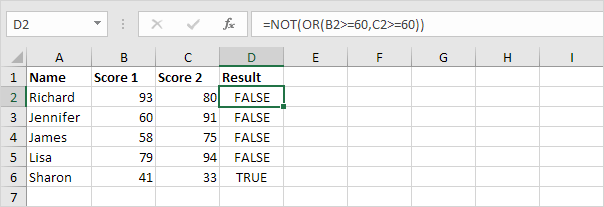# Logical Functions

If | And | Or | Not

Learn how to use Excel’s logical functions, such as IF, AND, OR and NOT.

### If

The IF function checks whether a condition is met, and returns one value if true and another value if false.

1. For example, take a look at the IF function in cell C2 below.Explanation: if the score is greater than or equal to 60, the IF function returns Pass, else it returns Fail. Visit our page about the IF function for many more examples.

### And

The AND Function returns TRUE if all conditions are true and returns FALSE if any of the conditions are false.

1. For example, take a look at the AND function in cell D2 below.Explanation: the AND function returns TRUE if the first score is greater than or equal to 60 and the second score is greater than or equal to 90, else it returns FALSE.

### Or

The OR function returns TRUE if any of the conditions are TRUE and returns FALSE if all conditions are false.

1. For example, take a look at the OR function in cell D2 below.Explanation: the OR function returns TRUE if at least one score is greater than or equal to 60, else it returns FALSE. Visit our page about the OR function for many more examples.

### Not

The NOT function changes TRUE to FALSE, and FALSE to TRUE.

1. For example, take a look at the NOT function in cell D2 below.Explanation: in this example, the NOT function reverses the result of the OR function (see previous example).